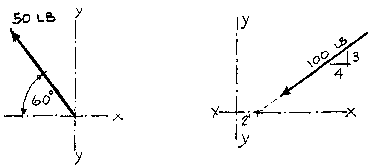## Lecture 8Example Problem

The Triangle Method
Given:
the force system shown below.
Determine:
the rectangular components Fx and Fy (H and V) by inspection and similar triangles; confirm this with a graphical solution. Note: Use a scale of 1" = 50 lb. (Drawings shown below are NOT to scale.)Solution:
Both forces belong to easily recognizable triangles.

The system on the left is given away by its 60 degree angle. It is a 1-2-sqrt3 triangle. Fx = 1/2 F or 25# and Fy = (sqrt3)/2 F or 43.5#

The system on the right can be solved by inspection realizing that the triangle is the familiar 3-4-5. So, 100/5 = Fy/3 = Fx/4; thus, Fy = 60# and Fx = 80#.

Both systems can be verified through strictly graphic methods. Carefully draw the force to scale and then the horizontal and vertical components in the "head to tail" fashion of the triangle method. These components are then simply measured. The accuracy of the results rely upon the accuracy of the drawing.